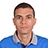Question

# The beta of a company is 1.51 while its financial leverage is 27%. What is then its unlevered beta if the corporate tax rate is 40%? $4 decimal places$

69

likes
345 views

## Answer to a math question The beta of a company is 1.51 while its financial leverage is 27%. What is then its unlevered beta if the corporate tax rate is 40%? $4 decimal places$Hank4.8
Le bêta d'une entreprise est de 1,51 tandis que son levier financier est de 27 %. Que le taux d'imposition des sociétés soit de 40 %. Pour trouver le bêta sans effet de levier, on peut utiliser la formule : \text = \frac}) \times \text} \texte \texte = \frac Simplifier : \texte = \frac \texte \texte = \frac Calcul du résultat : \texte \environ 1,2995 Ainsi, le bêta sans endettement de l’entreprise, avec un taux d’impôt sur les sociétés de 40 %, est d’environ 1,2995 $arrondi à quatre décimales$.
Frequently asked questions $FAQs$
Math question: Find the derivative of f$x$ = 5x^3 - 2x^2 + 4x - 7 with respect to x.
Question: Simplify the expression 2x$3x + 5$ - 4$x - 2$ using the distributive property.
Question: Find the area of a triangle with side lengths 5, 8, and 10 using Heron’s Formula. $Hint: s = (5+8+10$/2)